# Equation of circle 2

Find the equation of a circle that touches the axis of y at a distance of 4 from the origin and cuts off an intercept of length 6 on the axis x.

Result

f = (Correct answer is: )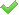### Step-by-step explanation: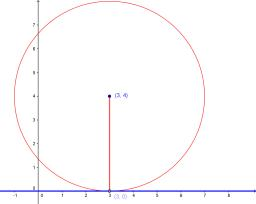Did you find an error or inaccuracy? Feel free to write us. Thank you!Tips to related online calculators
Line slope calculator is helpful for basic calculations in analytic geometry. The coordinates of two points in the plane calculate slope, normal and parametric line equation(s), slope, directional angle, direction vector, the length of the segment, intersections of the coordinate axes, etc.
Looking for help with calculating roots of a quadratic equation?
Do you have a linear equation or system of equations and looking for its solution? Or do you have a quadratic equation?
Pythagorean theorem is the base for the right triangle calculator.

#### You need to know the following knowledge to solve this word math problem:

We encourage you to watch this tutorial video on this math problem:

## Related math problems and questions:

• On lineOn line p: x = 4 + t, y = 3 + 2t, t is R, find point C, which has the same distance from points A [1,2] and B [-1,0].
• Center of line segmentCalculate the distance of the point X [1,3] from the center of the line segment x = 2-6t, y = 1-4t ; t is from interval <0,1>.
• Right triangle from axesA line segment has its ends on the coordinate axes and forms with them a triangle of area equal to 36 square units. The segment passes through the point ( 5,2). What is the slope of the line segment?
• Find the 5Find the equation of the circle with center at (1,20), which touches the line 8x+5y-19=0
• Touch x-axisFind the equations of circles that pass through points A (-2; 4) and B (0; 2) and touch the x-axis.
• Distance problemA=(x, x) B=(1,4) Distance AB=√5, find x;
• Sphere equationObtain the equation of sphere its centre on the line 3x+2z=0=4x-5y and passes through the points (0,-2,-4) and (2,-1,1).
• Isosceles triangleIn an isosceles triangle ABC with base AB; A [3,4]; B [1,6] and the vertex C lies on the line 5x - 6y - 16 = 0. Calculate the coordinates of vertex C.
• Tangent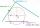What distance is the tangent t of the circle (S, 4 cm) and the chord of this circle, which is 6 cm long and parallel to the tangent t?
• Equation of circlefind an equation of the circle with indicated properties: a. center at (-3,5), diameter 20. b. center at origin and diameter 16.
• Chord BC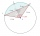A circle k has the center at the point S = [0; 0]. Point A = [40; 30] lies on the circle k. How long is the chord BC if the center P of this chord has the coordinates: [- 14; 0]?
• On a lineOn a line p : 3 x - 4 y - 3 = 0, determine the point C equidistant from points A[4, 4] and B[7, 1].
• Distance problem 2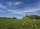A=(x,2x) B=(2x,1) Distance AB=√2, find value of x
• ChordIn a circle with radius r=60 cm is chord 4× longer than its distance from the center. What is the length of the chord?
• Find the 13Find the equation of the circle inscribed in the rhombus ABCD where A[1, -2], B[8, -3] and C[9, 4].
• Two chordsCalculate the length of chord AB and perpendicular chord BC to circle if AB is 4 cm from the center of the circle and BC 8 cm from the center of the circle.
• Find parametersFind parameters of the circle in the plane - coordinates of center and radius: ?